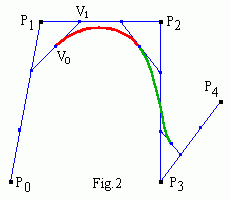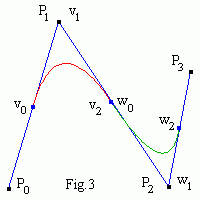# Building cubic B-spline

The problems with a single Bezier spline range from the need of a high degree curve to accurately fit a complex shape, which is inefficient to process. To overcome the problems, a piecewise curve is used. Therefore the order of the curve is not dependent on the number of control points.Earlier we built C1 continuous Cardinal spline. Now we will make a piecewise C2 continuous uniform B-spline of two cubic Bezier segments V(t) and W(t) with the control points (V0 , V1 , V2 , V3) and (W0 , W1 , W2 , W3) (see Fig.1). Recall that for cubic Bezier spline    P '(0) = 3(P1 - P0) ,    P '(1) = 3(P3 - P2) ,    P "(0) = 6(P0 - 2P1 + P2) ,    P "(1) = 6(P1 - 2P2 + P3) . Note also, that for any two points a and b the point (2a - b) = a + (a - b) is "mirror b with respect to a".
It is evident, that from B-spline continuity at the junction point W(0) = V(1) we get W0 = V3.
Continuity of the first derivative W '(0) = V '(1) leads to
W1 - W0 = V3 - V2   =>   W1 = 2V3 - V2
i.e. W1 is mirror V2 w.r.t. V3.
At last continuity of the second derivative W "(0) = V "(1) leads to
W0 - 2W1 + W2 = V1 - 2V2 + V3   =>   W2 = 2W1 - (2V2 - V1)
i.e. to get W2 first mirror V1 w.r.t. V2 then w.r.t. W1 .
We see, that only one control point W3 of the Bezier curve W(t) is really free. Therefore we need four control points for the first segment, then one more point per segment to set a B-spline. In general case the result is that a curve with n+1 control points is composed of n-k+2 segments, each of degree k-1.

From Fig.2 it follows, that one can express all control points of Bezier segments in terms of the deBoor points P0,1,...,n (the corners) , e.g.
V1 = P1 + (P2 - P1)/3 = (2P1 + P2)/3 ,
V0 = [(P0 + 2P1)/3 + (2P1 + P2)/3]/2 = (P0 + 4P1 + P2)/6 .Then Bezier segments points can be computed. But one can evaluate points of k-order B-spline directly by the Cox - beBoor algorithm similar to deCasteljau iterations
Pir = Pir-1 (t - ti)/(ti+k-r - ti) + Pi-1r-1 [1 - (t - ti)/(ti+k-r - ti)] ,
where ti are knots, which determine the start and end of each segment. Bezier segments have local coordinates
τi = (t - ti)/(ti+1 - ti),     0 < τi < 1 ,     ti < t < ti+1 .
Bezier and deBoor control points of quadratic B-spline are shown in Fig.3.
Note that I've found the Cox - beBoor formula in the Net and didn't check it (see Spline formulae standartization). I used basis function calculation instead.

Contents   Previous: Interpolating Cardinal splines   Next: B-spline basis functions
updated 8 August 2001